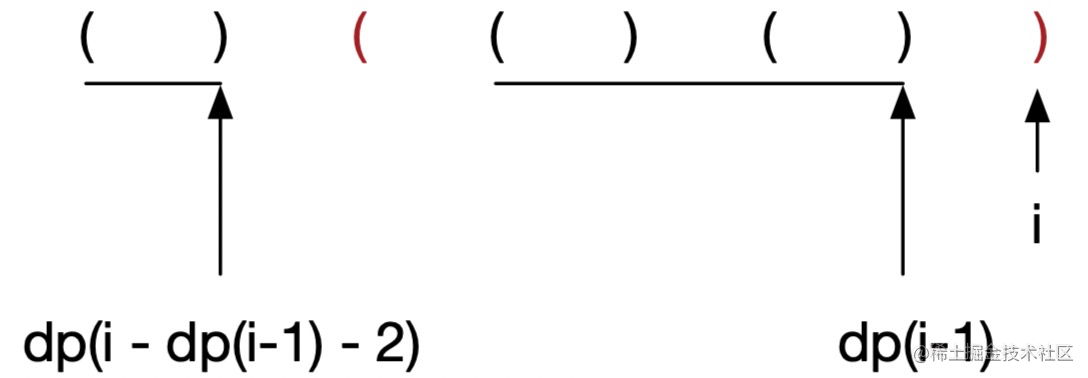# 精读《算法 - 动态规划》

1. 非常锻炼思维。动态规划是非常锻炼脑力的题目，虽然有套路，但每道题解法思路差异很大，作为思维练习非常合适。
2. 非常实用。动态规划听起来很高级，但实际上思路和解决的问题都很常见。

• 自动寻路哪种走法最优？
• 背包装哪些物品空间利用率最大？
• 怎么用最少的硬币凑零钱？

## 精读

1. 存在最优子结构。
2. 存在重复子问题。
3. 无后效性。

### 存在最优子结构

• 对于爬楼梯问题，由于每层台阶都是由前面台阶爬上来的，因此必然存在一个线性关系推导。
• 如果变成二维平面寻路呢？那么就升级为二维问题，存在两个变量 `i,j` 与上一步之间关系了。
• 如果是背包问题，同时存在物品数量 `i`、物品重量 `j` 和物品质量 `k` 三个变量呢？那就升级为三位问题，需要寻找三个之间的关系。

### 爬楼梯问题

• `dp(i) = dp(i-1) + dp(i-2)`
• `dp(1) = 1`
• `dp(2) = 2`

``````function dp(i: number) {
switch (i) {
case 1:
return 1;
case 2:
return 2;
default:
return dp(i - 1) + dp(i - 2);
}
}

return dp(n);

``````const cache: number[] = [];

function dp(i: number) {
switch (i) {
case 1:
cache[i] = 1;
break;
case 2:
cache[i] = 2;
break;
default:
cache[i] = cache[i - 1] + cache[i - 2];
}

return cache[i];
}

// 既然用了缓存，最好子底向上递归，这样前面的缓存才能优先算出来
for (let i = 1; i <= n; i++) {
dp(i);
}

return cache[n];

``````const cache: [number, number] = [];

function dp(i: number) {
switch (i) {
case 1:
cache[i % 2] = 1;
break;
case 2:
cache[i % 2] = 2;
break;
default:
cache[i % 2] = cache[(i - 1) % 2] + cache[(i - 2) % 2];
}

return cache[i % 2];
}

for (let i = 1; i <= n; i++) {
dp(i);
}

return cache[n % 2];

### 最大子序和

• `dp(i) = dp(i-1) + nums[i]` 如果 `dp(i-1) > 0`
• `dp(i) = nums[i]` 如果 `dp(i-1) <= 0`

### 最长递增子序列

`dp[i] = max(dp[j]) + 1`，其中 `0<=j<i``num[j]<num[i]`

### 最长有效括号

`dp(i) = dp(i-2) + 2`

`dp(i) = dp(i-1) + dp(i - dp(i-1) - 2) + 2`，你可以结合下面的图来理解：### 栅栏涂色

`k` 种颜色的涂料和一个包含 `n` 个栅栏柱的栅栏，每个栅栏柱可以用其中一种颜色进行上色。

### 编辑距离

• 插入一个字符
• 删除一个字符
• 替换一个字符

1. 假设是替换，即 `dp(i,j) = dp(i-1,j-1) + 1`，因为替换最后一个字符只要一步，并且和前面字符没什么关系，所以前面的最小操作次数直接加过来。
2. 假设是插入，即 `word1` 插入一个字符变成 `word2`，那么只要变换到这一步再 +1 插入操作就行了，变换到这一步由于插入一个就行了，因此 `word1``word2` 少一个单词，其它都一样，要变换到这一步，就要进行 `dp(i,j-1)` 的变换，因此 `dp(i,j) = dp(i,j-1) + 1`。。
3. 假设是删除，即 `word1` 删除一个字符变成 `word2`，同理，要进行 `dp(i-1,j)` 的变化后多一步删除，因此 `dp(i,j) = dp(i-1,j) + 1`

`i` 为 -1 时，即 `word1` 为空，此时要变换为 `word2` 很显然，只有插入 `j` 次是最小操作次数，因此此时 `dp(i,j) = j`；同理，当 `j` 为 -1 时，即 `word2` 为空，此时要删除 `i` 次，因此操作次数为 `i`，所以 `dp(i,j) = i`

## 总结